# Partial Derivative: Definition, Rules & Examples

Lesson Transcript
Instructor
Gerald Lemay

Gerald has taught engineering, math and science and has a doctorate in electrical engineering.

Expert Contributor
Robert Ferdinand

Robert Ferdinand has taught university-level mathematics, statistics and computer science from freshmen to senior level. Robert has a PhD in Applied Mathematics.

When a function depends on more than one variable, we can use the partial derivative to determine how that function changes with respect to one variable at a time. In this lesson, we use examples to define partial derivatives and to explain the rules for evaluating them. Updated: 09/15/2020

## Partial Derivative Definition

Calories consumed and calories burned have an impact on our weight. Let's say that our weight, u, depended on the calories from food eaten, x, and the amount of physical exertion we do, y. If we only regulated our eating, while doing the same exercise every day, we could ask how does u change when we vary only x. Likewise, we could keep x constant and take note of how u varies when we change y. This would be like keeping a constant daily diet while changing how much we exercise. This idea of change with respect to one variable while keeping other variables constant is at the heart of the partial derivative.

When we write u = u(x,y), we are saying that we have a function, u, which depends on two independent variables: x and y. We can consider the change in u with respect to either of these two independent variables by using the partial derivative. The partial derivative of u with respect to x is written as: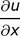What this means is to take the usual derivative, but only x will be the variable. All other variables will be treated as constants. We can also determine how u changes with y when x is held constant. This is the partial of u with respect to y. It's written as: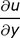For example, if u = y * x^2, then: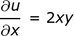Likewise, we can differentiate with respect to y and treat x as a constant with the equation: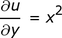The rule for partial derivatives is that we differentiate with respect to one variable while keeping all the other variables constant. As another example, find the partial derivatives of u with respect to x and with respect to y for: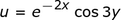To do this example, we will need the derivative of an exponential with the following: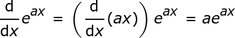And the derivative of a cosine, which is written as: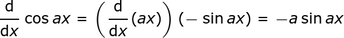Thus: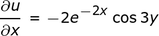and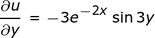An error occurred trying to load this video.

Try refreshing the page, or contact customer support.

Coming up next: Partial Differentiation: Definition, Rules & Application

### You're on a roll. Keep up the good work!

Replay
Your next lesson will play in 10 seconds
• 0:04 Partial Derivative Definition
• 3:04 Second Order Derivatives
• 7:06 Extending Partial Derivatives
• 8:23 Other Derivative Notations
• 9:00 Lesson Summary
Save Save

Want to watch this again later?

Log in or sign up to add this lesson to a Custom Course.

Timeline
Autoplay
Autoplay
Speed Speed Audio mode

## Second Order Derivatives

So far we have defined and given examples for first-order partial derivatives. Second-order partial derivatives are simply the partial derivative of a first-order partial derivative. We can have four second-order partial derivatives, which you can see right here: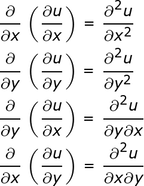Continuing with our first example of u = y * x^2,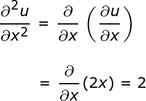and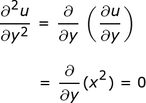Likewise,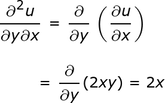and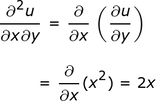And in our second example: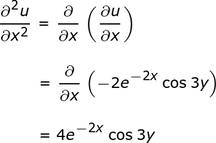and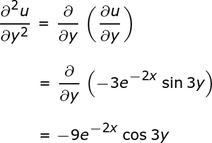The mixed partial derivatives become: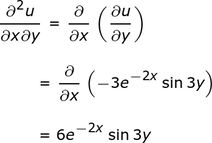and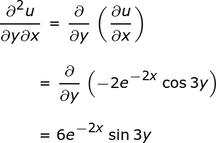To unlock this lesson you must be a Study.com Member.
Create your account

Additional Activities

## Partial Derivative Practice Questions

1. The function f(x, y) gives us the profit (in dollars) of a certain commodity as the number of commodities x sold and the number of days y the commodity is on the market. The function is below:

f(x, y) = (x^2) e^(-y).

Find the rate of change of the profit with respect to the number of commodities sold and the number of days the commodity stays on the market.'

2. The following function below is a product of a logarithmic function and a trigonometric function. Find its first-order, partial derivatives:

g(x, y) = ln(x) cos(4y).

### Answers

1. To find the rate of change of the profit, f, with respect to the number commodities sold, x, we take the partial derivative of f with respect to x while keeping y as constant. This yields the following:

f_x = e^(-y) (x^2)' = e^(-y) 2x = 2x e^(-y) dollars per number of commodities sold.

Similarly, to find the rate of change of the profit, f, with respect to the number of days, y, that the commodity stays on the market, we calculate the partial derivative of f with respect to y while keeping x as constant. This yields the following:

f_y = [e^(-y)]' (x^2) = -e^(-y) (x^2) = -(x^2) e^(-y) dollars per day.

2. To get the first-order, partial derivative of g(x, y) with respect to x, we differentiate g with respect to x, while keeping y constant. This leads to the following, first-order, partial derivative:

g_x = [ln(x)]' cos(4y) = (1/x) cos(4y).

Similarly, to get the first-order, partial derivative of g(x, y) with respect to y, we differentiate g with respect to y, while keeping x constant. This leads to the following, first-order, partial derivative:

g_y = ln(x) [cos(4y)]' = ln(x) -4 sin(4y) = -4 ln(x) sin(4y).

### Register to view this lesson

Are you a student or a teacher?

### Unlock Your Education

#### See for yourself why 30 million people use Study.com

##### Become a Study.com member and start learning now.
Back
What teachers are saying about Study.com
Create an account to start this course today
Used by over 30 million students worldwide